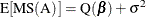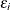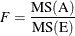### Tests of Effects Based on Expected Mean Squares

Statistical tests in analysis of variance models can be constructed by comparing independent mean squares. To test a particular null hypothesis, you compute the ratio of two mean squares that have the same expected value under that hypothesis; if the ratio is much larger than 1, then that constitutes significant evidence against the null. In particular, in an analysis of variance model with fixed effects only, the expected value of each mean square has two components: quadratic functions of fixed parameters and random variation. For example, for a fixed effect called A, the expected value of its mean square iswhereis the common variance of the.

Under the null hypothesis of no A effect, the fixed portion Q() of the expected mean square is zero. This mean square is then compared to another mean square—say, MS(E)—that is independent of the first and has the expected value. The ratio of the two mean squareshas an F distribution under the null hypothesis.

When the null hypothesis is false, the numerator term has a larger expected value, but the expected value of the denominator remains the same. Thus, large F values lead to rejection of the null hypothesis. The probability of getting an F value at least as large as the one observed given that the null hypothesis is true is called the significance probability value (or the p-value). A p-value of less than 0.05, for example, indicates that data with no A effect will yield F values as large as the one observed less than 5% of the time. This is usually considered moderate evidence that there is a real A effect. Smaller p-values constitute even stronger evidence. Larger p-values indicate that the effect of interest is less than random noise. In this case, you can conclude either that there is no effect at all or that you do not have enough data to detect the differences being tested.

The actual pattern in expected mean squares of terms related to fixed quantities (Q()) and functions of variance components depends on which terms in your model are fixed effects and which terms are random effects. This has bearing on how F statistics can be constructed. In some instances, exact tests are not available, such as when a linear combination of expected mean squares is necessary to form a proper denominator for an F test and a Satterthwaite approximation is used to determine the degrees of freedom of the approximation. The GLM and MIXED procedures can generate tables of expected mean squares and compute degrees of freedom by Satterthwaite’s method. The MIXED and GLIMMIX procedures can apply Satterthwaite approximations and other degrees-of-freedom computations more widely than in analysis of variance models. See the section Fixed, Random, and Mixed Models in Chapter 3: Introduction to Statistical Modeling with SAS/STAT Software, for a discussion of fixed versus random effects in statistical models.